# Chapter 10 Correlation and Regression We deal with

• Slides: 48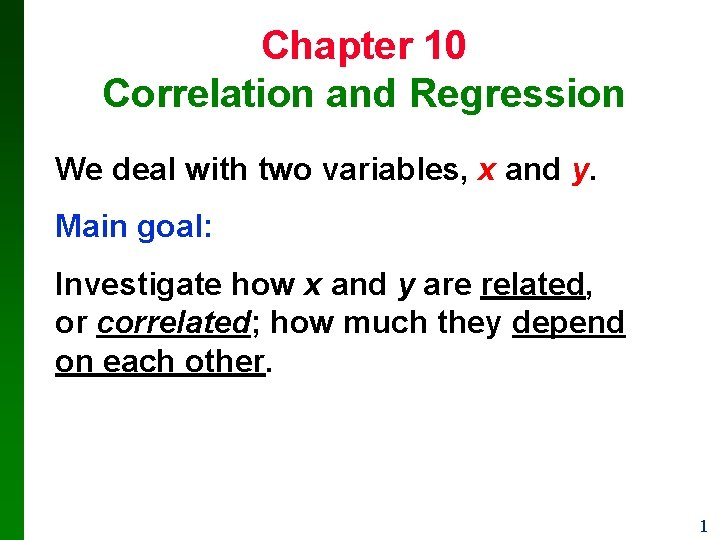Chapter 10 Correlation and Regression We deal with two variables, x and y. Main goal: Investigate how x and y are related, or correlated; how much they depend on each other. 1Example • x is the height of mother • y is the height of daughter Question: are the heights of daughters independent of the height of their mothers? Or is there a correlation between the heights of mothers and those of daughters? If yes, how strong is it? 2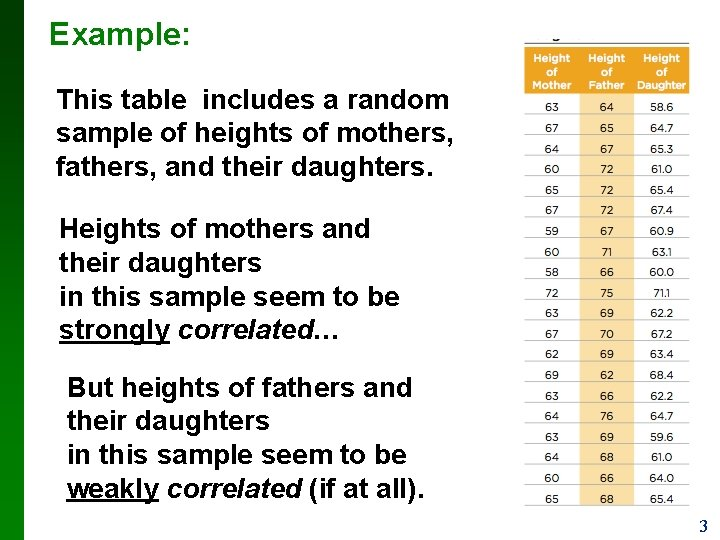Example: This table includes a random sample of heights of mothers, fathers, and their daughters. Heights of mothers and their daughters in this sample seem to be strongly correlated… But heights of fathers and their daughters in this sample seem to be weakly correlated (if at all). 3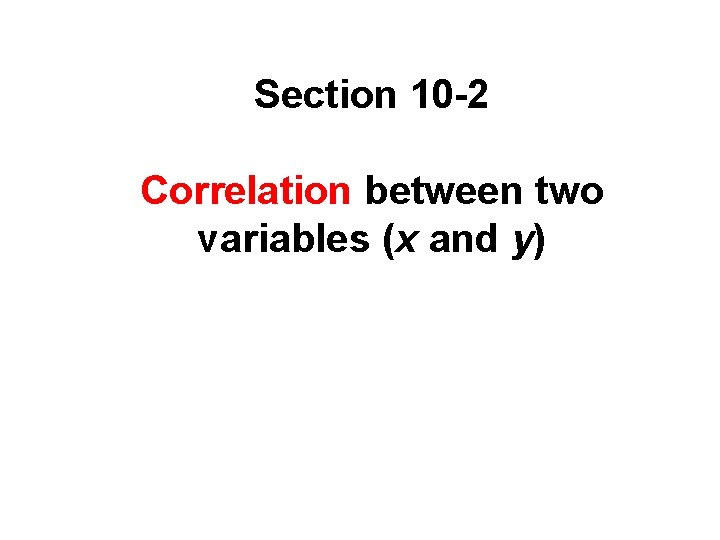Section 10 -2 Correlation between two variables (x and y) 4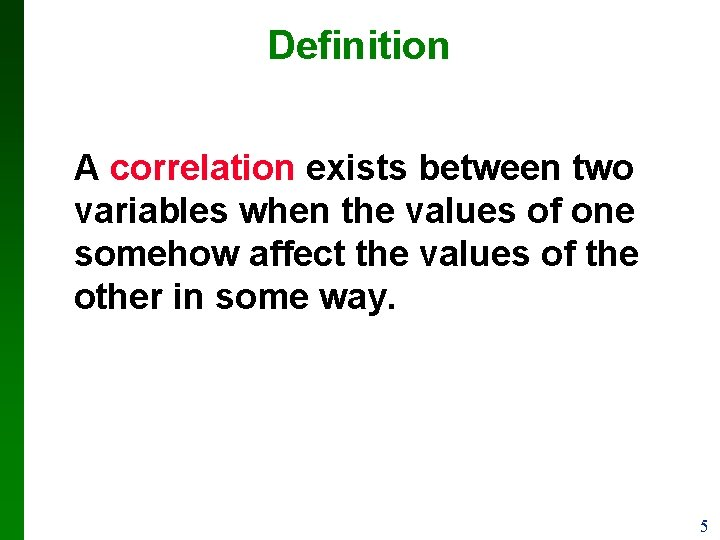Definition A correlation exists between two variables when the values of one somehow affect the values of the other in some way. 5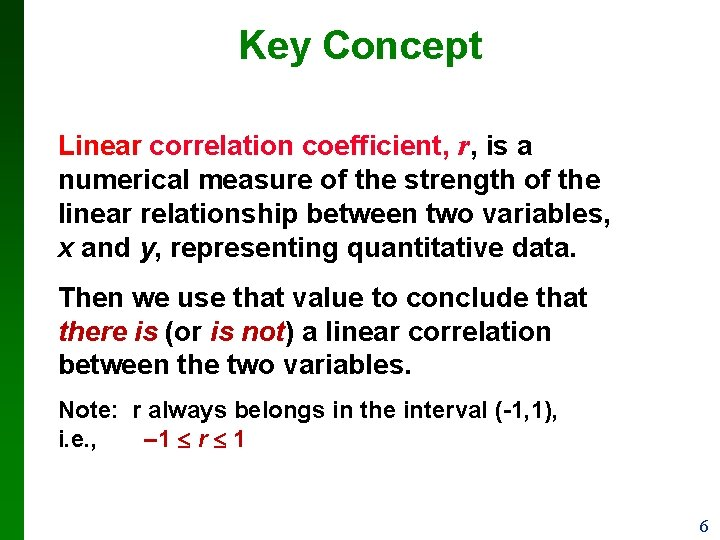Key Concept Linear correlation coefficient, r, is a numerical measure of the strength of the linear relationship between two variables, x and y, representing quantitative data. Then we use that value to conclude that there is (or is not) a linear correlation between the two variables. Note: r always belongs in the interval (-1, 1), i. e. , – 1 r 1 6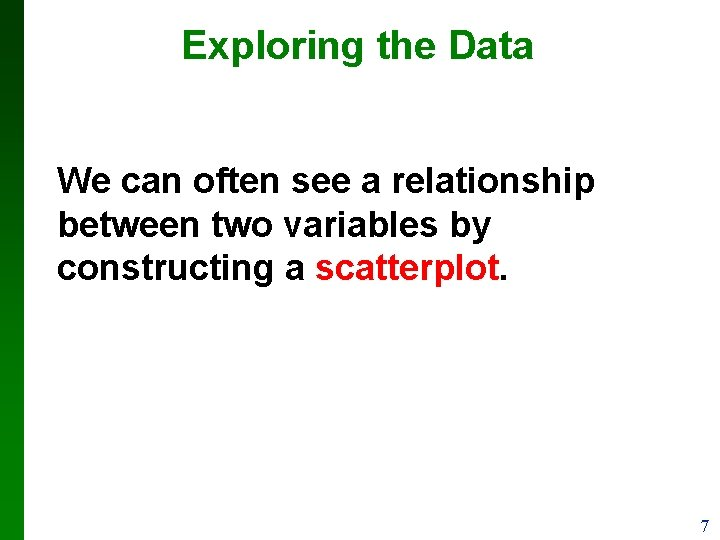Exploring the Data We can often see a relationship between two variables by constructing a scatterplot. 7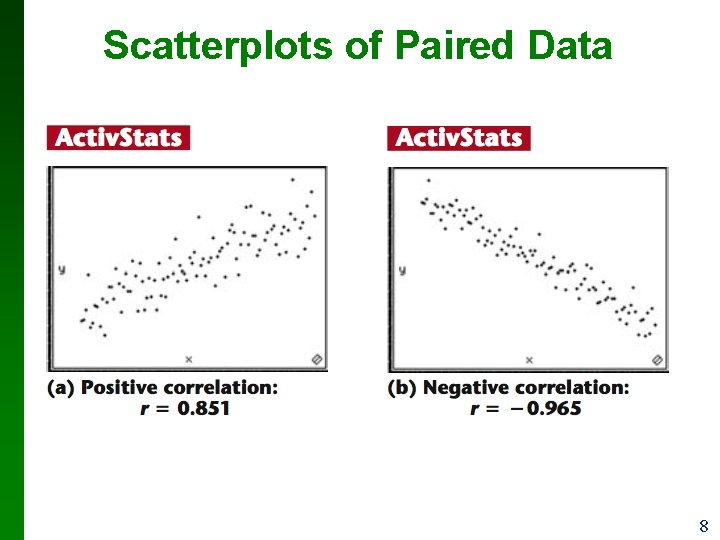Scatterplots of Paired Data 8Scatterplots of Paired Data 9Scatterplots of Paired Data 10Requirements 1. The sample of paired (x, y) data is a random sample of quantitative data. 2. Visual examination of the scatterplot must confirm that the points approximate a straight-line pattern. 3. The outliers must be removed if they are known to be errors. (We will not do that in this course…) 11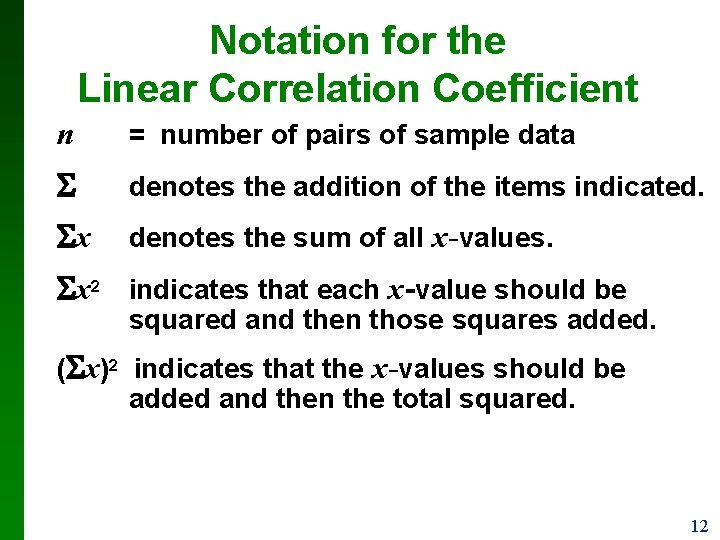Notation for the Linear Correlation Coefficient n = number of pairs of sample data denotes the addition of the items indicated. x denotes the sum of all x-values. x 2 indicates that each x-value should be squared and then those squares added. ( x)2 indicates that the x-values should be added and then the total squared. 12Notation for the Linear Correlation Coefficient xy indicates that each x-value should be first multiplied by its corresponding y-value. After obtaining all such products, find their sum. r = linear correlation coefficient for sample data. = linear correlation coefficient for population data, i. e. linear correlation between two populations. 13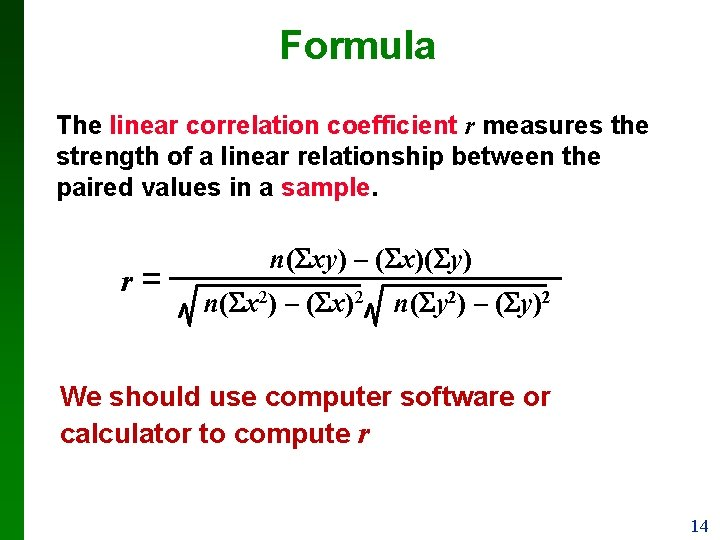Formula The linear correlation coefficient r measures the strength of a linear relationship between the paired values in a sample. r= n( xy) – ( x)( y) n( x 2) – ( x)2 n( y 2) – ( y)2 We should use computer software or calculator to compute r 14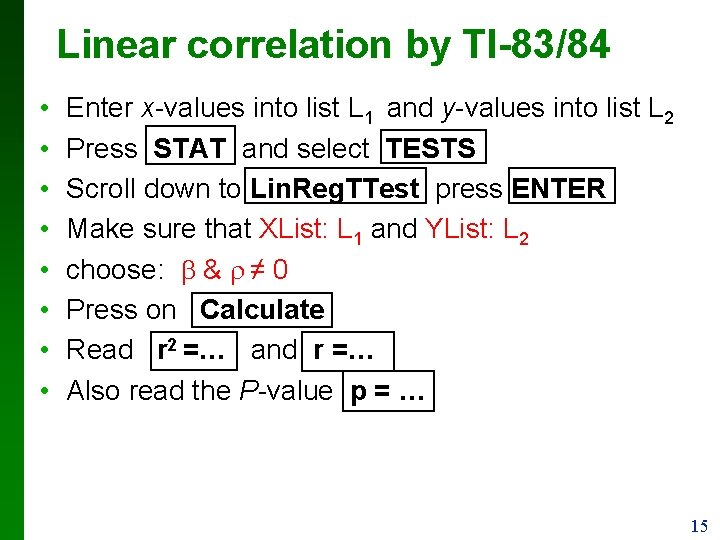Linear correlation by TI-83/84 • • Enter x-values into list L 1 and y-values into list L 2 Press STAT and select TESTS Scroll down to Lin. Reg. TTest press ENTER Make sure that XList: L 1 and YList: L 2 choose: b & r ≠ 0 Press on Calculate Read r 2 =… and r =… Also read the P-value p = … 15Interpreting r Using Table A-6: If the absolute value of the computed value of r, denoted |r|, exceeds the value in Table A-6, conclude that there is a linear correlation. Otherwise, there is not sufficient evidence to support the conclusion of a linear correlation. Note: In most cases we use the significance level a = 0. 05 (the middle column of Table A-6). 16Interpreting r Using P-value computed by calculator: If the P-value is ≤ a, conclude that there is a linear correlation. Otherwise, there is not sufficient evidence to support the conclusion of a linear correlation. Note: In most cases we use the significance level a = 0. 05. 17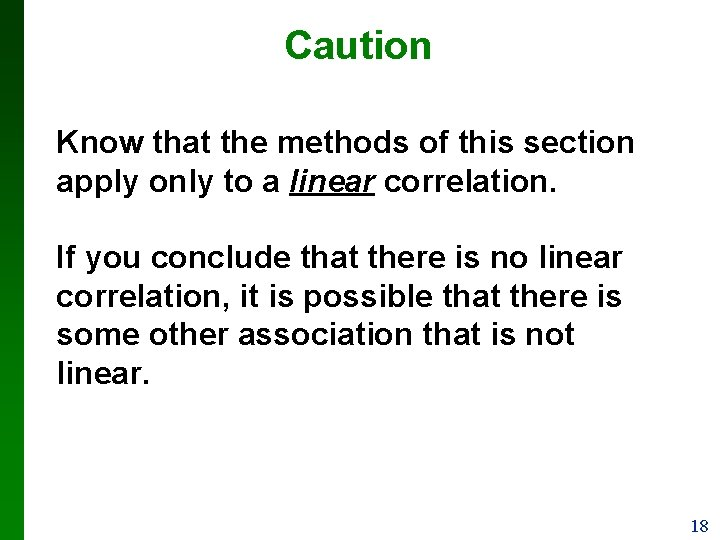Caution Know that the methods of this section apply only to a linear correlation. If you conclude that there is no linear correlation, it is possible that there is some other association that is not linear. 18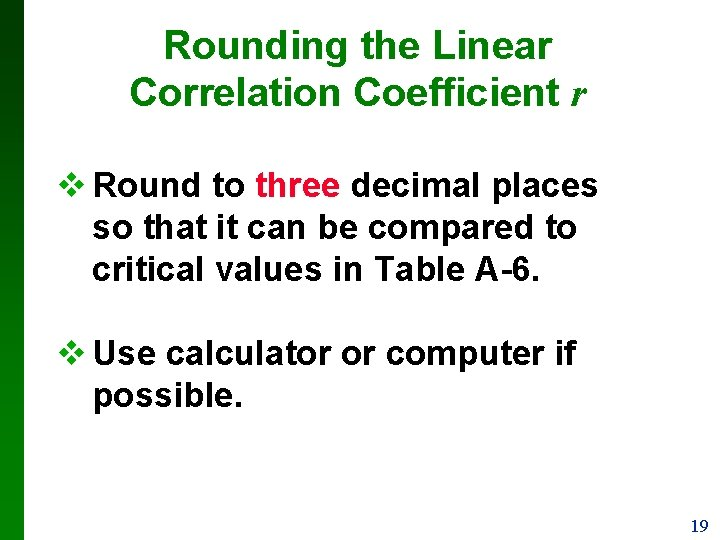Rounding the Linear Correlation Coefficient r v Round to three decimal places so that it can be compared to critical values in Table A-6. v Use calculator or computer if possible. 19Properties of the Linear Correlation Coefficient r 1. – 1 r 1 2. if all values of either variable are converted to a different scale, the value of r does not change. 3. The value of r is not affected by the choice of x and y. Interchange all x- and y-values and the value of r will not change. 4. r measures strength of a linear relationship. 5. r is very sensitive to outliers, they can dramatically affect its value. 20Example: The paired pizza/subway fare costs from Table 10 -1 are shown here in a scatterplot. Find the value of the linear correlation coefficient r for the paired sample data. 21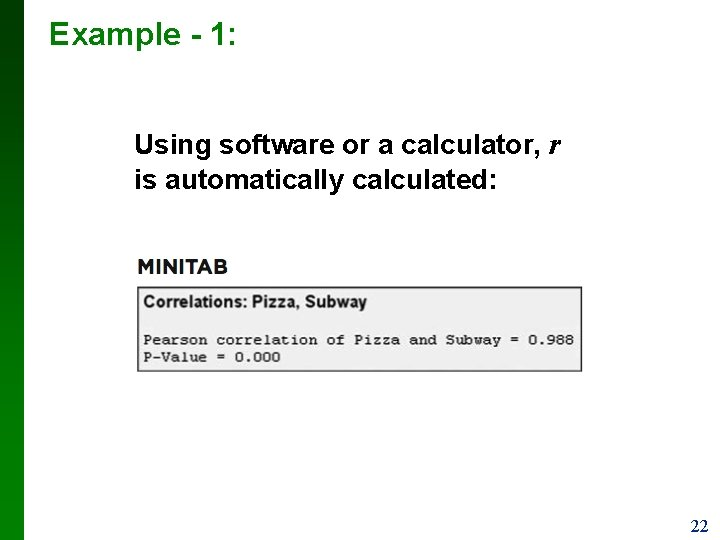Example - 1: Using software or a calculator, r is automatically calculated: 22Interpreting the Linear Correlation Coefficient r Critical Values from Table A-6 and the Computed Value of r 23Example - 2: Using a 0. 05 significance level, interpret the value of r = 0. 117 found using the 62 pairs of weights of discarded paper and glass listed in Data Set 22 in Appendix B. Is there sufficient evidence to support a claim of a linear correlation between the weights of discarded paper and glass? 24Example: Using Table A-6 to Interpret r: If we refer to Table A-6 with n = 62 pairs of sample data, we obtain the critical value of 0. 254 (approximately) for = 0. 05. Because |0. 117| does not exceed the value of 0. 254 from Table A-6, we conclude that there is not sufficient evidence to support a claim of a linear correlation between weights of discarded paper and glass. 25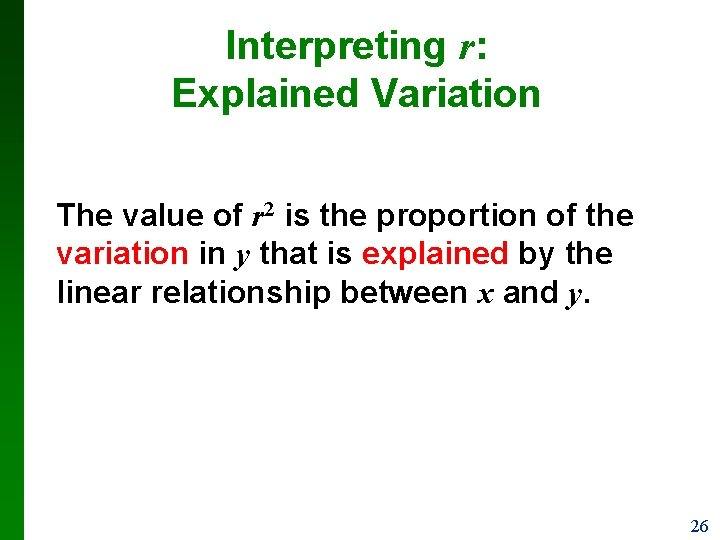Interpreting r: Explained Variation The value of r 2 is the proportion of the variation in y that is explained by the linear relationship between x and y. 26Example: Using the pizza subway fare costs, we have found that the linear correlation coefficient is r = 0. 988. What proportion of the variation in the subway fare can be explained by the variation in the costs of a slice of pizza? With r = 0. 988, we get r 2 = 0. 976. We conclude that 0. 976 (or about 98%) of the variation in the cost of a subway fares can be explained by the linear relationship between the costs of pizza and subway fares. This implies that about 2% of the variation in costs of subway fares cannot be explained by the costs of pizza. 27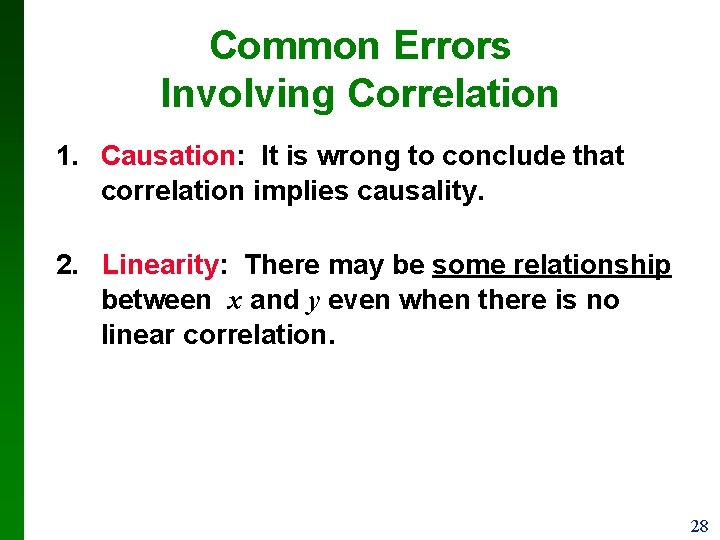Common Errors Involving Correlation 1. Causation: It is wrong to conclude that correlation implies causality. 2. Linearity: There may be some relationship between x and y even when there is no linear correlation. 28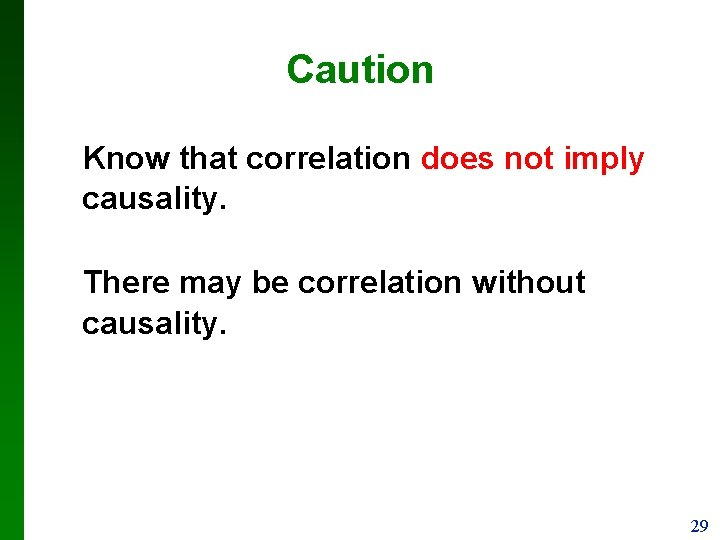Caution Know that correlation does not imply causality. There may be correlation without causality. 29Section 10 -3 Regression 30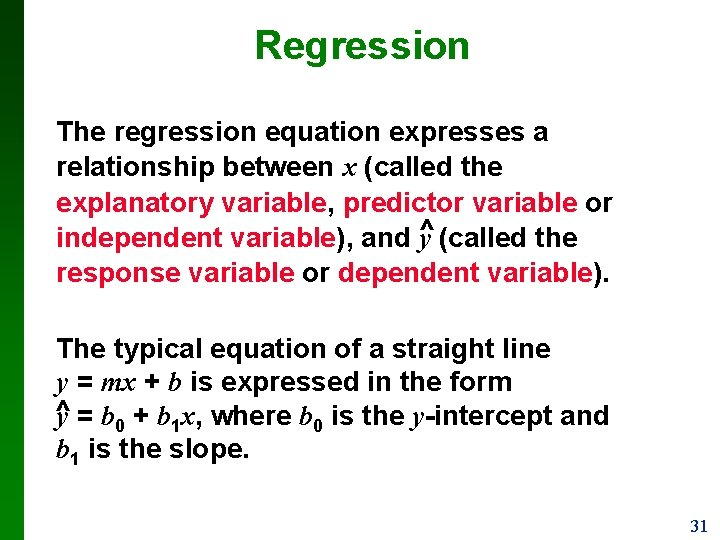Regression The regression equation expresses a relationship between x (called the explanatory variable, predictor variable or ^ independent variable), and y (called the response variable or dependent variable). The typical equation of a straight line y = mx + b is expressed in the form ^ y = b 0 + b 1 x, where b 0 is the y-intercept and b 1 is the slope. 31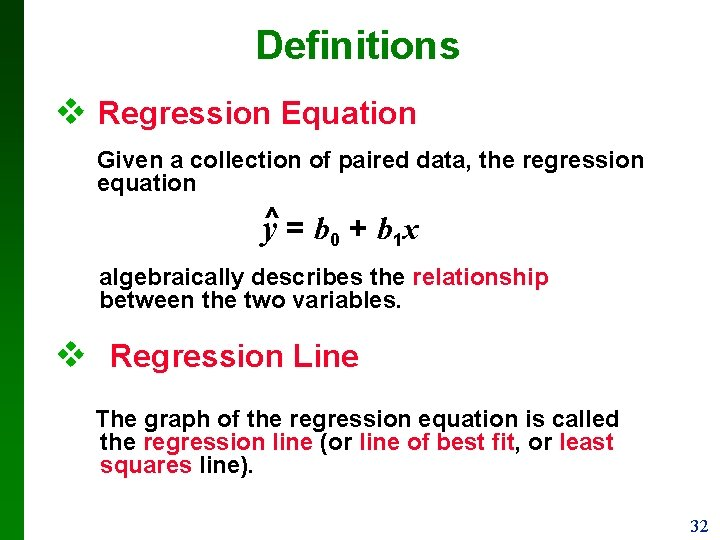Definitions v Regression Equation Given a collection of paired data, the regression equation y^ = b 0 + b 1 x algebraically describes the relationship between the two variables. v Regression Line The graph of the regression equation is called the regression line (or line of best fit, or least squares line). 32Example: 33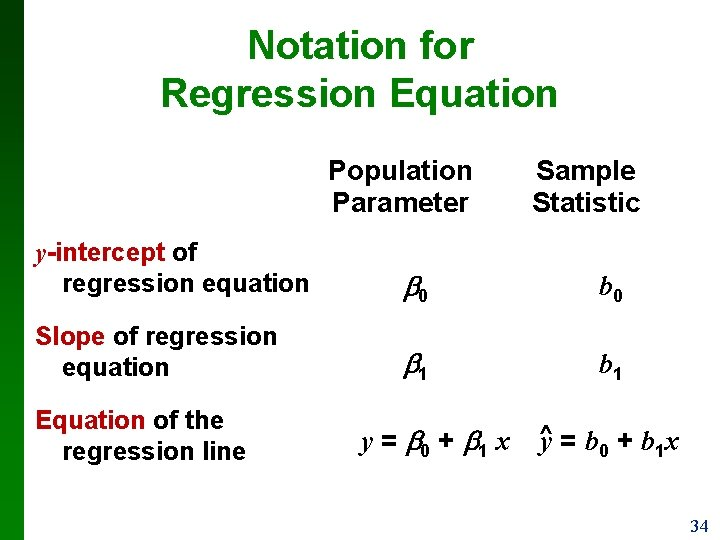Notation for Regression Equation Population Parameter Sample Statistic y-intercept of regression equation 0 b 0 Slope of regression equation 1 b 1 Equation of the regression line y = 0 + 1 x ^y = b 0 + b 1 x 34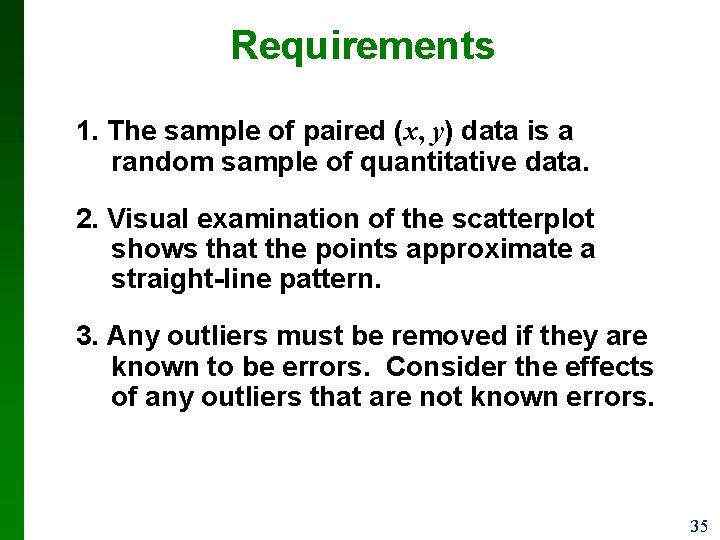Requirements 1. The sample of paired (x, y) data is a random sample of quantitative data. 2. Visual examination of the scatterplot shows that the points approximate a straight-line pattern. 3. Any outliers must be removed if they are known to be errors. Consider the effects of any outliers that are not known errors. 35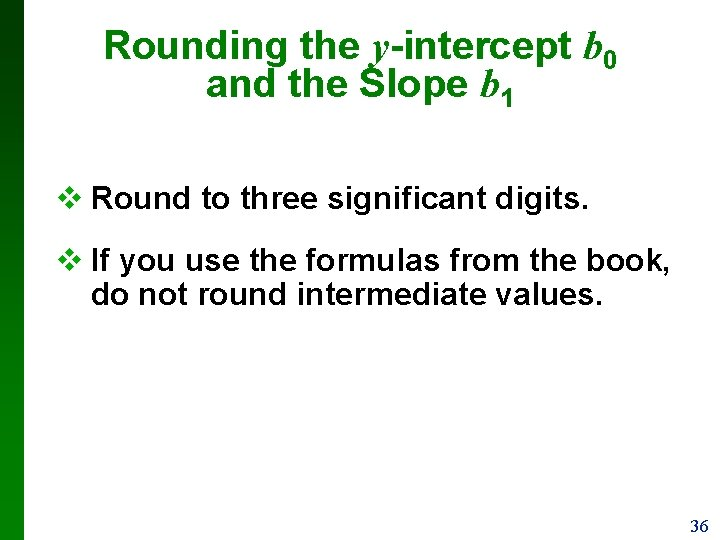Rounding the y-intercept b 0 and the Slope b 1 v Round to three significant digits. v If you use the formulas from the book, do not round intermediate values. 36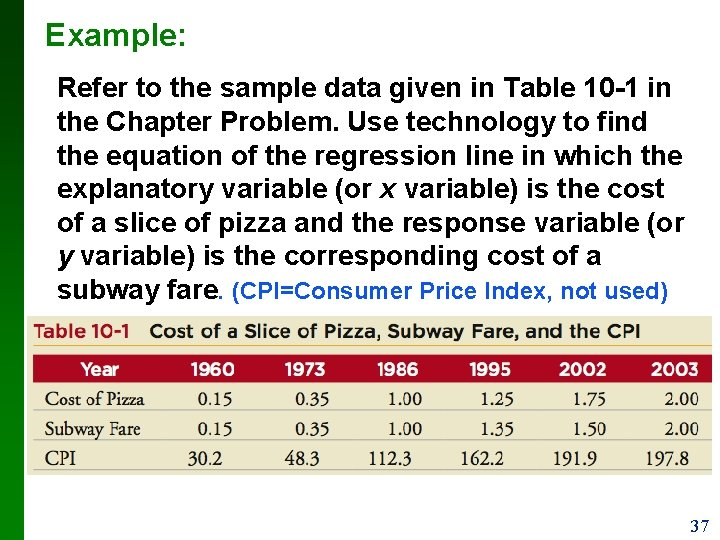Example: Refer to the sample data given in Table 10 -1 in the Chapter Problem. Use technology to find the equation of the regression line in which the explanatory variable (or x variable) is the cost of a slice of pizza and the response variable (or y variable) is the corresponding cost of a subway fare. (CPI=Consumer Price Index, not used) 37Example: Requirements are satisfied: simple random sample; scatterplot approximates a straight line; no outliers Here are results from four different technologies 38Example: Note: in TI-83/84, a means b 0 and b means b 1 All of these technologies show that the regression equation is ^ y = 0. 0346 +0. 945 x, ^ where y is the predicted cost of a subway fare and x is the cost of a slice of pizza. We should know that the regression equation is an estimate of the true regression equation. 39Example: Graph the regression equation (from the preceding Example) on the scatterplot of the pizza/subway fare data and examine the graph to subjectively determine how well the regression line fits the data. 40Example: 41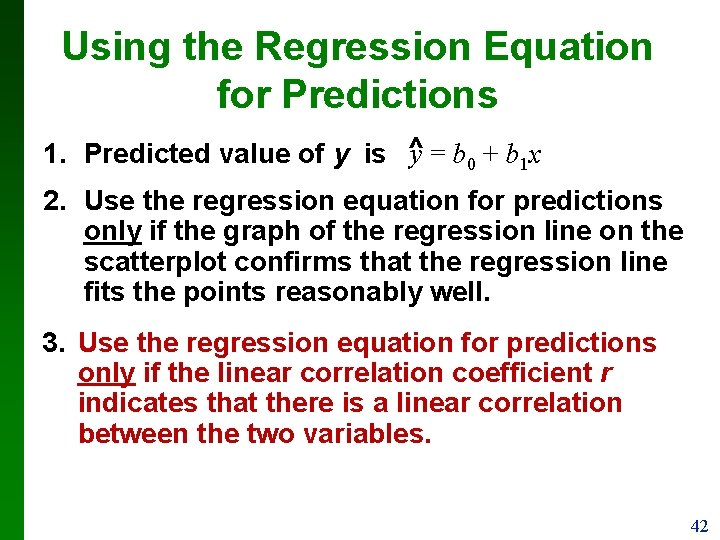Using the Regression Equation for Predictions ^ = b 0 + b 1 x 1. Predicted value of y is y 2. Use the regression equation for predictions only if the graph of the regression line on the scatterplot confirms that the regression line fits the points reasonably well. 3. Use the regression equation for predictions only if the linear correlation coefficient r indicates that there is a linear correlation between the two variables. 42Using the Regression Equation for Predictions 4. Use the regression line for predictions only if the value of x does not go much beyond the scope of the available sample data. (Predicting too far beyond the scope of the available sample data is called extrapolation, and it could result in bad predictions. ) 5. If the regression equation does not appear to be useful for making predictions, the best predicted value of a variable is its point _ estimate, which is its sample mean, y. 43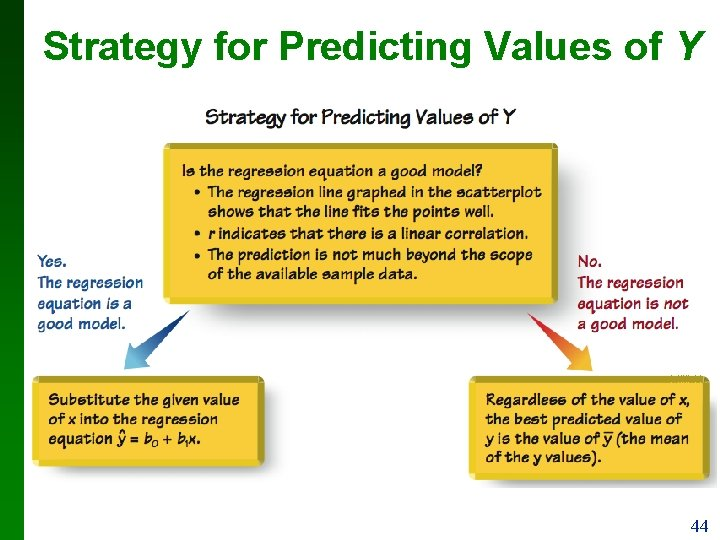Strategy for Predicting Values of Y 44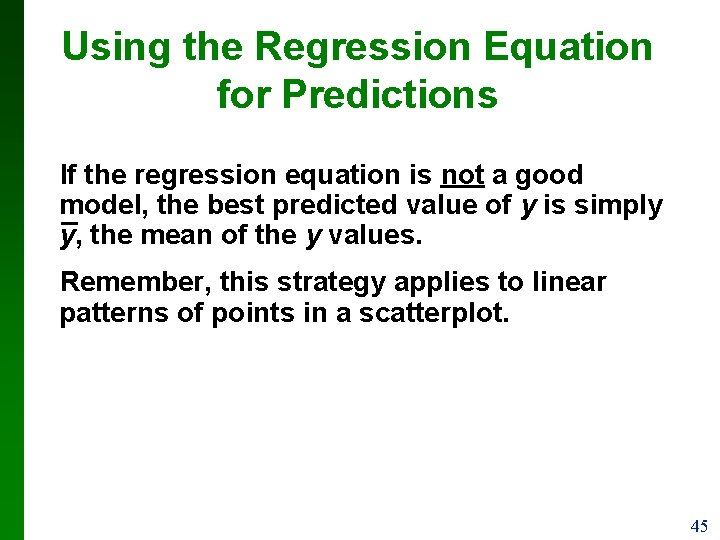Using the Regression Equation for Predictions If the regression equation is not a good model, the best predicted value of y is simply _ y, the mean of the y values. Remember, this strategy applies to linear patterns of points in a scatterplot. 45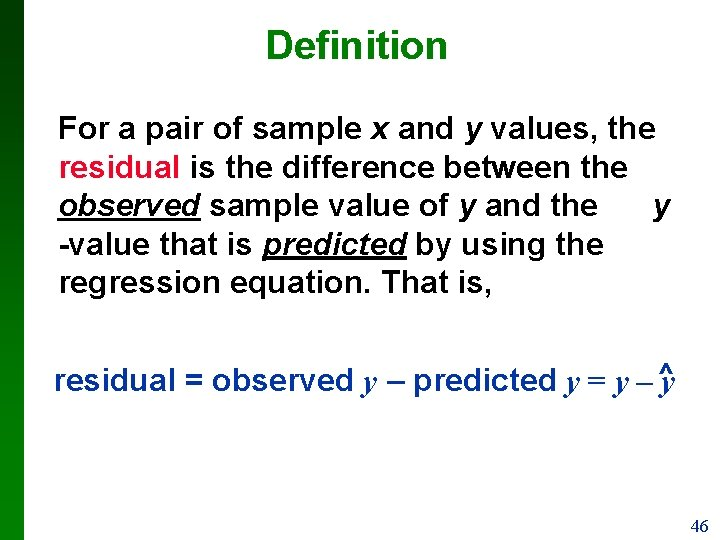Definition For a pair of sample x and y values, the residual is the difference between the observed sample value of y and the y -value that is predicted by using the regression equation. That is, = y – ^y residual = observed y – predicted y 46Residuals 47Definition A straight line satisfies the least-squares property if the sum of the squares of the residuals is the smallest sum possible. 48